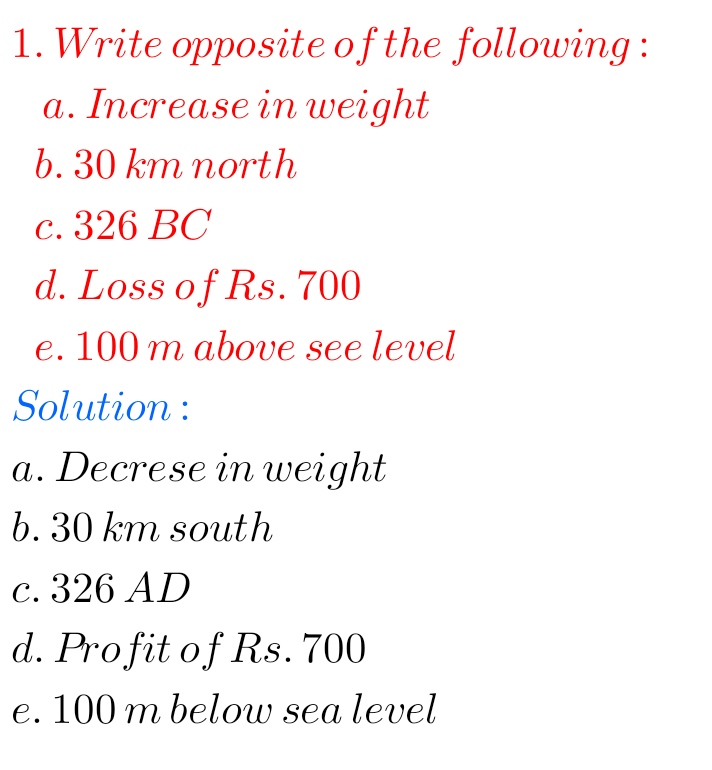## NCERT solutions for class 6th maths chapter 6/Integers/solutions for Integers, problems with solutions of Integers

NCERT SOLUTIONS FOR CLASS 6TH MATHS CHAPTER 6, INTEGERS NCERT class 6 maths chapter 6 Integers exercise 6.1 solutions are given. You should study the textbook lesson Integers very well. Observe the solutions and try them in your own method. Class 6 maths solutions NCERT Chapter 6 Integers Exercise 6.1 F F F F Note …